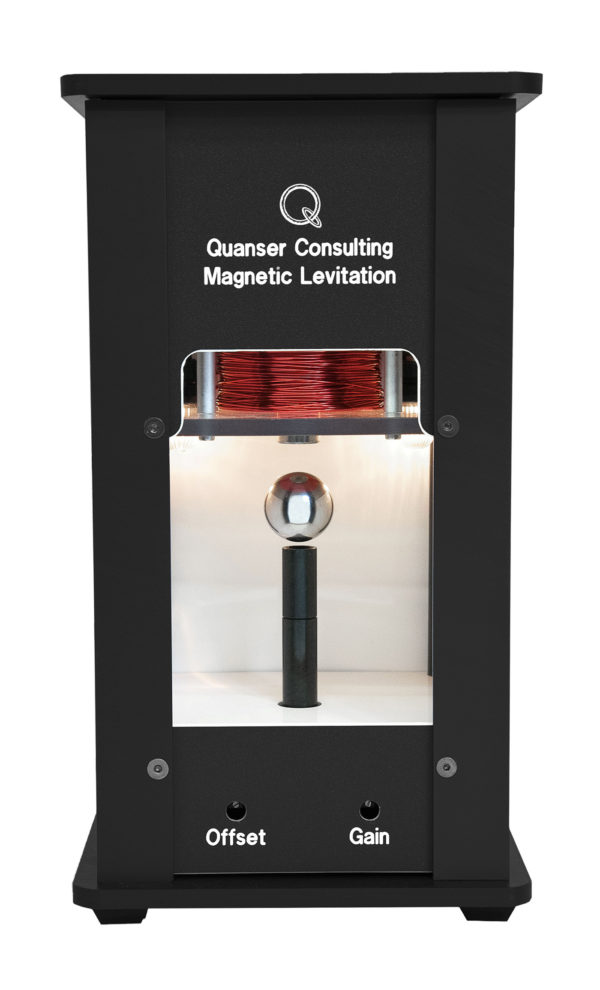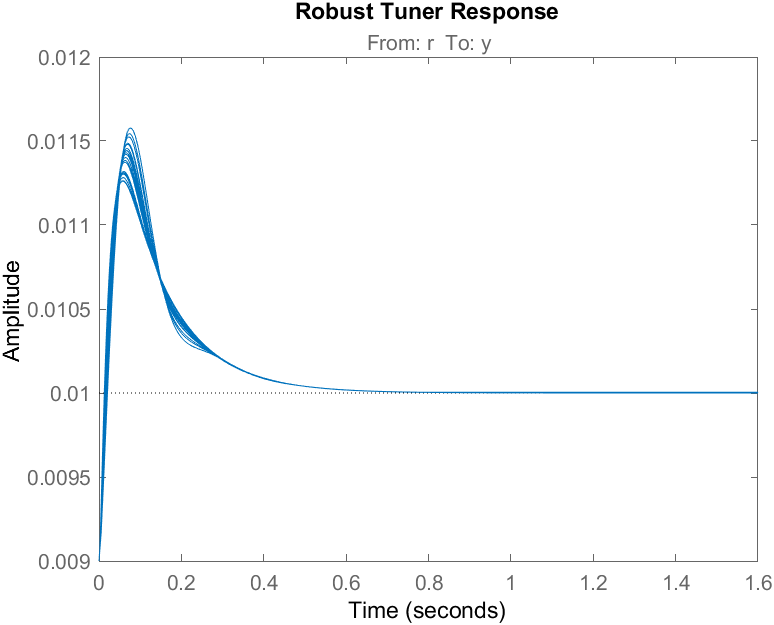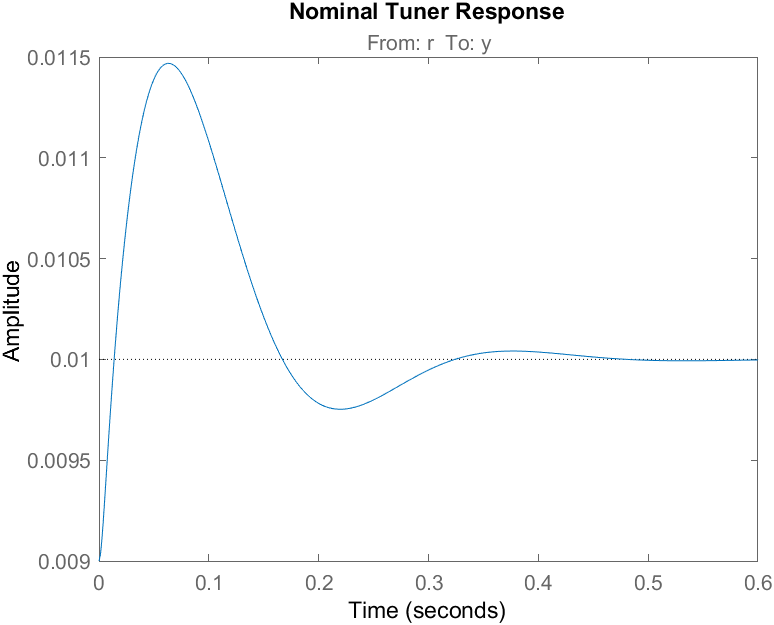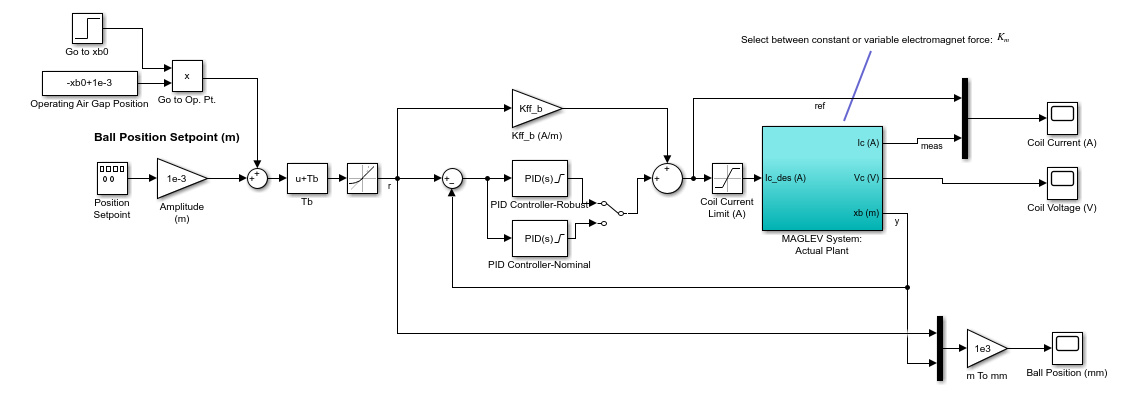## Robust Control for the Quanser Magnetic Levitation System

Version 1.0.0 (5.14 MB) by
Design and simulate the robust ball position control for the Quanser Magnetic Levitation System.

Updated 4 Mar 2022

Leveraging the MathWorks® Robust Control Toolbox for the Quanser Magnetic Levitation
The Quanser Magnetic Levitation shown below is a one-dimensional magnetic levitation system. It has an optical ball position sensor and a current sensor to measure the current in the electromagnet coils.Given the inherit nonlinear dynamics and uncertainties, robust control techniques are often used in magnetic levitation and other similar applications. Here we show how to use the MathWorks® Robust Control Toolbox™ to define an uncertain variable and use the Control System Tuner app to tune a robust controller that can help compensate for the variance in the magnet force constant and the other uncertainties that are not accounted for.
Before You Run this Example
The robust control can be simulated in MATLAB and Simulink using a model of the Magnetic Levitation system. Also, if you have the actual Quanser Magnetic Levitation hardware then you run the control on that system as well. The following toolboxes and additional software are required depending on what you want to do:
1. MathWorks Control System Toolbox to define transfer functions in MATLAB and so on.
2. MathWorks Simulink Control Design Toolbox. Used to linearize the nonlinear model and design the control gains with the Control System Tuner app.
3. MathWorks Robust Control Toolbox. Required to define uncertain parameters that is used when performing the robust control tuning.
4. [optional] Quanser QUARC Real-Time Control Software is required to run the controllers on the actual Magnetic Levitation hardware. If you don’t have the QUARC software, you can sign up for free trial.
How Does it Work?
The control design is discussed in this Quanser blog and documented in the Maglev_Robust_Tuning Live Script posted. The step responses of the nominal and robust controllers are simulated in MATLAB directly in the Maglev_Robust_Tuning Live Script. Sample responses are shown below. Because the robust controller uses the uncertain model, it shows the responses for different values of the electromagnet force constant. The nominal control is based on the nominal model with a fixed electromagnet constant and is used as a reference point.The more realistic nonlinear model-based simulation can be performed in the s_maglev_pid.slx Simulink model shown below.How to Run the Example
1. Go through the Maglev_Robust_Tuning.mlx Live Script posted. It guides you through the robust control process and simulates it directly in the Live Script.
2. Simulate the ball position control in the s_maglev_pid.slx Simulink model posted using either the nominal or robust control gains. You can select between the two controllers using the Manual Switch.
If you have the Quanser Magnetic Levitation hardware available, then you can run the nominal and robust controllers on the system using the q_maglev_pid_nominal.slx and q_maglev_pid_robust.slx Simulink models with the Quanser QUARC Real-Time Control. To do this:
1. Make sure the Quanser Magnetic Levitation system has been setup (and tested using the Quick Start Guide).
2. The sensors should be calibrated and the VoltPAQ amplifier powered and set to a Gain of 3.
3. Run the Maglev_Robust_Tuning Live Script up to the “Robust Control Implementation” section.
4. Open the q_maglev_pid_robust.slx Simulink model.
5. If you are not using the Quanser Q2-USB data-acquisition device, then change the Board type in the HIL Initialize block found in the MAGLEV w/ PI Control | MAGLEVsubsystem.
6. Click on the Monitor & Tune button in the Simulnk model Hardware tab to generate and run the QUARC real-time code. The ball should start levitation.
7. Once the ball is stabilized at a fixed point, you can add a square wave command by setting the Amplitude (m) Gain block to 1e-3 (for 1 mm) or something larger.
8. Click on the Stop button to stop running the controller.
9. The new measured results can be plotted in Live Script.
10. Follow a similar procedure to implement nominal control gain with q_maglev_pid_nominal.slx.

### Cite As

Quanser (2023). Robust Control for the Quanser Magnetic Levitation System (https://www.mathworks.com/matlabcentral/fileexchange/107534-robust-control-for-the-quanser-magnetic-levitation-system), MATLAB Central File Exchange. Retrieved .

##### MATLAB Release Compatibility
Created with R2021a
Compatible with any release
##### Platform Compatibility
Windows macOS Linux